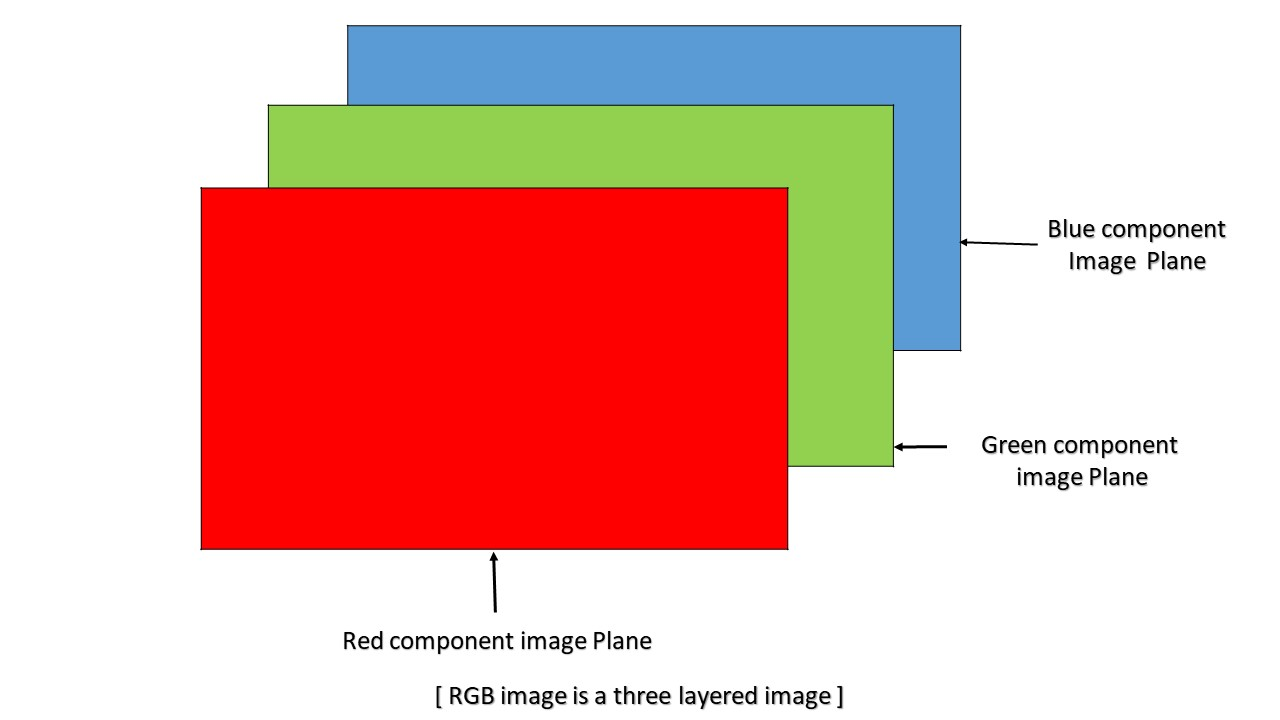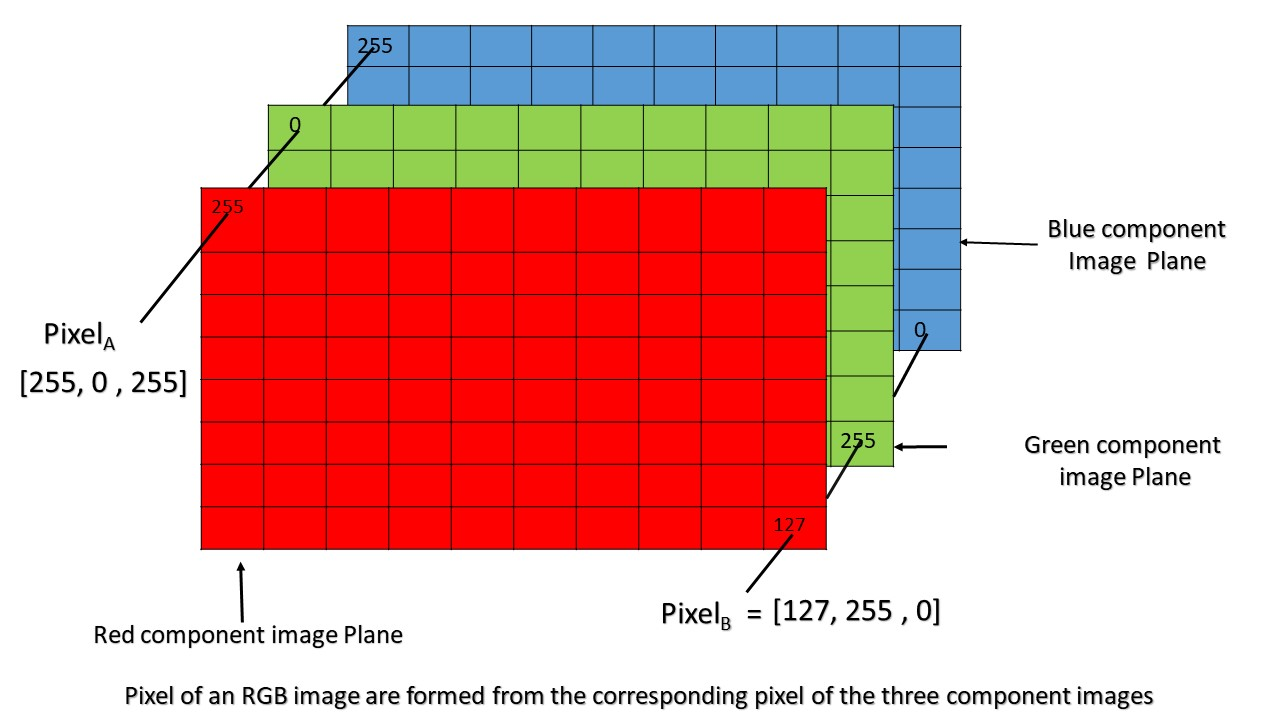# MATLAB | RGB image representation

An RGB image can be viewed as three different images(a red scale image, a green scale image and a blue scale image) stacked on top of each other, and when fed into the red, green and blue inputs of a colour monitor, it produces a colour image on the screen.

An RGB image is sometimes referred to as a true colour image as the precision with which a real-life image can be replicated has led to the nickname “true colour image.”In MATLAB, an RGB image is basically a M*N*3 array of colour pixel, where each colour pixel is associated with three values which correspond to red, blue and green colour component of RGB image at a specified spatial location.
So, the colour of any pixel is determined by the combination of the red, green, and blue intensities stored in each colour plane at the pixel’s location.
Here each colour plane is a M*N array.As can be seen in the above image, Pixel(A) has value (255, 0, 255) and is determined by the combination of intensities stored in the red colour plane, green colour plane and blue colour plane respectively.

Similarly, pixel(B) has value (127, 255, 0) and is determined in the same manner as pixel(A).

Colour planes of RGB image:
Consider an RGB image array ‘I’ then,
I(:, :, 1) represents the Red colour plane of the RGB image
I(:, :, 2) represents the Green colour plane of the RGB image
I(:, :, 3) represents the Blue colour plane of the RGB image

RGB image array range:
In MATLAB, an RGB image array can be of class ‘double’, ‘uint8’, or ‘uint16’ datatype. The datatype class of colour component determines the range of values.
For example,
if an RGB image is of class ‘double’ then each colour component is a value between 0 and 1.
Similarly, if an RGB image is of class ‘uint8’, the range of values that each colour component can have is [0 – 255] and [0 – 65535 ] if the RGB image is of class ‘uint16’.

Bit depth:
The number of bits used to store a pixel value of component image determines the bit depth of an RGB image. For example, if each colour component image is an 8-bit image, the RGB image will be said to have 24 bit deep.

Possible number of colours in RGB image:
Let an RGB image is of class ‘uint8’, i.e the range of values a colour component plane can have is [0 – 255 ] ( a total of 256 shades of that colour).
So, each individual colour plane of An RGB image is capable of showing 256 shade of that colour.
So total number of combination of colour that can be represented in an RGB image is 256 X 256 X 256 = 16777216, approximately 16 million.

My Personal Notes arrow_drop_upCheck out this Author's contributed articles.

If you like GeeksforGeeks and would like to contribute, you can also write an article using contribute.geeksforgeeks.org or mail your article to contribute@geeksforgeeks.org. See your article appearing on the GeeksforGeeks main page and help other Geeks.

Please Improve this article if you find anything incorrect by clicking on the "Improve Article" button below.

Article Tags :

1

Please write to us at contribute@geeksforgeeks.org to report any issue with the above content.